# KSEEB Solutions for Class 7 Maths Chapter 12 Algebraic Expressions Ex 12.1

Students can Download Chapter 12 Algebraic Expressions Ex 12.1, Question and Answers, Notes Pdf, KSEEB Solutions for Class 7 Maths, Karnataka State Board Solutions help you to revise complete Syllabus and score more marks in your examinations.

## Karnataka State Syllabus Class 7 Maths Chapter 12 Algebraic Expressions Ex 12.1

Question 1.
Get the algebraic expressions in the following cases using variables, constants and arithmetic operations.
i) Subtraction of z from y.
Solution:
y – z

ii) One-half of the sum of numbers x and
Solution: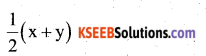iii) The number z multiplied by itself.
Solution:
Z × Z = Z2

iv) One- fourth of the product of numbers p and q.
Solution: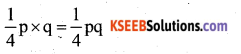v) Numbers x and y both squared and added.
Solution:
x2 + y2

vi) Number 5 added to three times the product of numbers m and n.
Solution:
3mn + 5

vii) Product of numbers y and z subtracted from 10.
Solution:
10 – yz

viii) Sum of numbers a and b subtracted from their product.
Solution:
ab, – {a + b)Question 2.
i) Identify the terms and their factors in the following expression show the terms and factors by tree diagrams.
Solution: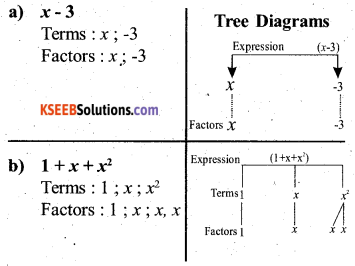c) y – y3
Terms: y; -y3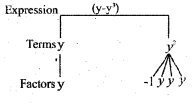Factors : y ; -1, y,

d) 5xy2 + 7x2y
Terms : 5xy2 ; 7x2y
Factors : 5, x, y, y; 7, x, x, y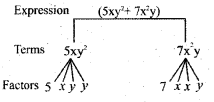e) -ab + 2b2 – 3a2
Terms : -ab ; 2b2; 3a2
Factors : 1, a, b ; 2, b, b ; -3, a, a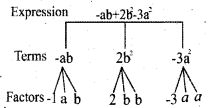ii) Identify terms and factors in the expressions given below :

a) -4x + 5
Terms : -4x ; 5
Factors : -4, x; 5

b) -4x + 5y
Terms : -4x ; 5y
Factors :-4, x; 5, yc) 5y + 3y2
Terms : 5y ; 3y2
Factors : 5, y ; 3, y, y

d) xy + 2x2y2
Terms : xy ; 2x2y2
Factors : x, y ; 2, x, x, y, y

e) pq + q
Terms : pq ; q
Factors : p, q ; q

f) 1.2 ab – 2.4b + 3.6a
Terms : 1.2 ab ; 2.4b ; 3.6a
Factors : 1.2, a, b ; -2.4, b ; 3.6, a

g)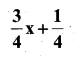Solution: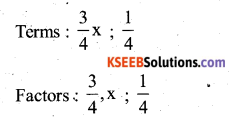h) 0.1 p2 + 0.2 q2
Terms : 0.1p2; 0.2q2
Factors : 0.1, p, p ; 0.2, q, q

Question 3.
Identify the numerical coefficients of terms (other than constants) in the following expressions :
i) 5 – 3t2
Term other than constant = -3/2
numerical co-efficient = -3

ii) 1 + t + t2 + t3
Term other than constant = t, t2, t3
Numerical co-efficients = 1, 1, 1.

iii) x + 2xy + 3y
Terms other than constants = x, 2xy + 3y
Numerical co-efficients = 1, 2, 3

iv) 100m + 1000n
Terms other than constants = 100m, 1000n
Numerical co-efficients = 100, 1000.v) -p2q2 + 7pq
Terms other than constants = -p2 q2, 7 pq
Numerical co-efficients = -1, 7

vi) 1.2a + 0.8b
Terms other than constants = 1.2a, 0.8b
Numerical co-efficients = 1.2, 0.8

vii) 3.14 r2
Terms other than constants = 3.14r2
Numerical co-efficients = 3.14

viii) 2 (l + b)
Terms other than constants = 2l, 2b
(∵ 2(l + b) = 2l + 2b)
Numerical co-efficients = 2, 2

ix) 0.1 y + 0.01 y2
Terms other than constants = 0.1 y, 0.01 y2
Numerical co-efficients = 0.1, 0.01Question 4.
a) Identify terms which contain x and give the coefficient of x.
i) y2x + y
Terms which contains x — y2
co-efficient of x — y2

ii) 13y2 – 8yx
Terms which contains x — (x – 8yx)
co-efficient of x — (-8y)

iii) x + y + 2
Terms which contains x —- x
co-efficient of x —- 1

iv) 5 + z + zx
Terms which contains x — x
co-efficient of x — z

v) 1 + x + xy
Terms which contains x — x and xy
co-efficient of x — 1 y

vi) 12xy2 + 25 — 12xy2
Terms which contains x
co-efficient of x — 12y2

vii) 7x + xy2
7x Terms which contains x
co-efficient of x —- 7
Terms which contains x — xy2
y2 co-efficient of

b) Identify terms which contain y2 and give the coefficient of y’.
i) 8 – xy2
Terms which contains y2
xy2 co-efficient of y2 — x

ii) 5y2 + 7x
Terms which contains y2
co-efficient of y2 — 5

iii) 2x2 y – 15xy2 + 7y2
Terms which contains y2 — (-15xy2)
co-efficient of y2 — (-15x)
Terms which contain y2 — 7y2
co-efficient of y2 —- 7Question 5.
Classify into monomials, binomials and trinomials.
i) 4y – 7z
This expression is binomial because it contains two terms. That is 4 y and -7z.
ii) y2
This is monomial expression because it contains only one term y2.
iii) x + y – xy
This is trinomial expression because it contains three terms x, y and -xy.
iv) 100
This is a monomial expression because it contains only one term 100.
v) ab – a – b
This is trinomial expression because it contains 3 terms ab, -a and -b.
vi) 5 – 3t
This is binomial expression because it contains 2 terms 5 and -3t.
vii) 4p2q – 4pq2
This is binomial expression because it contains two terms 4p2q and – 4 pq2
viii) 7mn
This is monomial expression because it contains only one term 7mn.
ix) z2 – 3z + 8
This is trinomial expression because it contains three terms z2, -3z and 8.
x) a2 + b2
This is binomial expression because it contains two terms a2 and b2.
xi) z2 + z
This is binomial expression because it contains 2 terms z2 and z.
xii) 1 + x + x2
This is trinomial expression because it contains 3 terms 1, x and x2.Question 6.
State whether a given pair of terms is of like or unlike terms.
i) 1, 100
like terms
ii)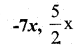like terms
iii) -29x, -29y
unlike terms
iv) 14xy, 42yx
like terms
v) 4m2p, 4mp2
unlike terms
vi) 12xz, 12x2z2 unlike terms

Question 7.
Identify like terms in the following :
a) -xy2, -4yx2, 8x2, 2xy2, 7y, – 11x2, 100x, – 11yx, 20x2y, -6x2, y, 2xy, 3x
Solutions:
Like terms are
i) -xy2, 2xy2
ii) -4yx2, 20x2y
iii) 8x2, -11x2, -6x2
iv) 7y, y
v) -100x, 3x
vi) -11yx, 2xy

b) 10pq, 7p, 8q, -p2q2, -7qp, – 100q, -23, 12q2p2, -5p2, 41, 2405p, 75qp, 13p2q, qp2, 701p2
Solution:
like terms are
i) 10pq, – 7qp, 78qp,
ii) 7p, 2405p,
iii) 8q, -100q
iv) -23, 41,
v) 12q2p2, -p2q2,
vi) -5p2, 701p2,
vii) 13p2q, qp2